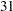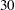## Example 57.1 Reading Means and Standard Errors from Variables in a DATA= Data Set

This example creates an ordinary SAS data set that contains sample means and standard errors computed from imputed data sets. These estimates are then combined to generate valid univariate inferences about the population means.

The following statements use the UNIVARIATE procedure to generate sample means and standard errors for the variables in each imputed data set:

```proc univariate data=outmi noprint;
var Oxygen RunTime RunPulse;
output out=outuni mean=Oxygen RunTime RunPulse
stderr=SOxygen SRunTime SRunPulse;
by _Imputation_;
run;
```

The following statements display the output data set from PROC UNIVARIATE shown in Output 57.1.1:

```proc print data=outuni;
title 'UNIVARIATE Means and Standard Errors';
run;
```

Output 57.1.1 UNIVARIATE Output Data Set
 UNIVARIATE Means and Standard Errors

Obs _Imputation_ Oxygen RunTime RunPulse SOxygen SRunTime SRunPulse
1 1 47.0120 10.4441 171.216 0.95984 0.28520 1.59910
2 2 47.2407 10.5040 171.244 0.93540 0.26661 1.75638
3 3 47.4995 10.5922 171.909 1.00766 0.26302 1.85795
4 4 47.1485 10.5279 171.146 0.95439 0.26405 1.75011
5 5 47.0042 10.4913 172.072 0.96528 0.27275 1.84807

The following statements combine the means and standard errors from imputed data sets, The EDF= option requests that the adjusted degrees of freedom be used in the analysis. For sample means based onobservations, the complete-data error degrees of freedom is.

```proc mianalyze data=outuni edf=30;
modeleffects Oxygen RunTime RunPulse;
stderr SOxygen SRunTime SRunPulse;
run;
```

The "Model Information" table in Output 57.1.2 lists the input data set(s) and the number of imputations. The "Variance Information" table in Output 57.1.2 displays the between-imputation variance, within-imputation variance, and total variance for each univariate inference. It also displays the degrees of freedom for the total variance. The relative increase in variance due to missing values, the fraction of missing information, and the relative efficiency for each imputed variable are also displayed. A detailed description of these statistics is provided in the section Combining Inferences from Imputed Data Sets and the section Multiple Imputation Efficiency.

Output 57.1.2 Variance Information
The MIANALYZE Procedure

Model Information
Data Set WORK.OUTUNI
Number of Imputations 5

Variance Information
Parameter Variance DF Relative
Increase
in Variance
Fraction
Missing
Information
Relative
Efficiency
Between Within Total
Oxygen 0.041478 0.930853 0.980626 26.298 0.053471 0.051977 0.989712
RunTime 0.002948 0.073142 0.076679 26.503 0.048365 0.047147 0.990659
RunPulse 0.191086 3.114442 3.343744 25.463 0.073626 0.070759 0.986046

The "Parameter Estimates" table in Output 57.1.3 displays the estimated mean and corresponding standard error for each variable. The table also displays a 95% confidence interval for the mean and a t statistic with the associated p-value for testing the hypothesis that the mean is equal to the value specified. You can use the THETA0= option to specify the value for the null hypothesis, which is zero by default. The table also displays the minimum and maximum parameter estimates from the imputed data sets.

Output 57.1.3 Parameter Estimates
Parameter Estimates
Parameter Estimate Std Error 95% Confidence Limits DF Minimum Maximum Theta0 t for H0:
Parameter=Theta0
Pr > |t|
Oxygen 47.180993 0.990266 45.1466 49.2154 26.298 47.004201 47.499541 0 47.64 <.0001
RunTime 10.511906 0.276910 9.9432 11.0806 26.503 10.444149 10.592244 0 37.96 <.0001
RunPulse 171.517500 1.828591 167.7549 175.2801 25.463 171.146171 172.071730 0 93.80 <.0001

Note that the results in this example could also have been obtained with the MI procedure.